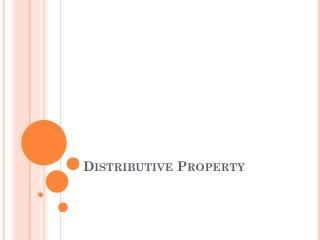DownloadDownload PresentationDistributive Property

# Distributive Property

Télécharger la présentation## Distributive Property

- - - - - - - - - - - - - - - - - - - - - - - - - - - E N D - - - - - - - - - - - - - - - - - - - - - - - - - - -
##### Presentation Transcript

1. Distributive Property

2. To multiply a sum or difference, multiply each number within the parentheses by the number outside the parentheses. 3(2x + 6) = 3(2x) + 3(6) = 6x+18 (x + 2)3 = x(3) + 2(3) = 3x+6 6(7x – 4) = 6( 7x) – 6( 4) = 42x-24 (3x – 1)6 = 3x( 6) -1( 6 ) =18x-6

3. -9(2 – 8y) = =-9( 2) – (-9)( 8y) Distributive Property. = -18 - (-72y) Multiply = -18+ 72y Simplify (5m + 6)11 = 5m(11) + 6(11) = 55m+ 66